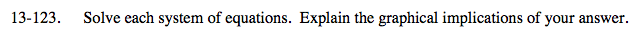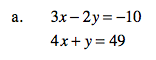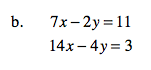### Home > A2C > Chapter 13 > Lesson 13.2.2 > Problem13-123

13-123.(8, 17)

What does this mean about the graphs of the two equations?Multiply the first equation by (−2) and add the two equations.